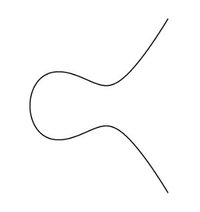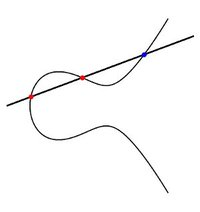Because of the attack on the computer network of the University of Duisburg-Essen, some content (in particular some image files) cannot be accessed because it is stored on central servers of the university.

# Research

It is not easy to present our research topics briefly and in a way accessible to non-mathematicians. Below, you find the beginnings of an attempt to do so …

If you can read German, we also recommend the following three articles written by members of the Essen Seminar:

Massimo Bertolini, Kubische Kurven

Jochen Heinloth, Higgsbündel

Jan Kohlhaase, Kammern, Apartments, Gebäude

The basic theme of algebraic geometry is the study of solutions of a system of polynomial equations in geometric terms. For example, the solution of the equation $x^2+y^2=1$ in the $(x,y)$-plane is precisely the circle around the origin with radius 1 – a geometric object.Figure 1

As a second example we consider the equation $y^2=x^2(x+1)$, whose solution set is shown in Figure 1. An easily described geometric property of this “curve with a node” is that, upon magnification, the curve looks like a straight line at all points except at the origin where it looks like two intersecting lines. We call the origin a singular point of the curve and the other points, smooth points.Figure 2 Figure 3

Equations such as that of the elliptic curve are also interesting from the number theoretic point of view – in this case we are primarily interested in solutions of the equation in the set of integers or rational numbers. It turns out that the “geometric methods” can still be used quite profitably. The interaction between algebraic geometry and number theory works in both directions. It dominates a large part of modern number theory and algebraic geometry has given important impetus.

Themes that play especially important roles in the Essen seminar are:

Parameter spaces and moduli spaces: Here, one attempts to classify all objects of a certain kind (for example all elliptic curves) by another geometric object, thereby providing a geometric structure to this class of objects. For instance, the set of elliptic curves can be understood (in a suitable sense) in a natural way as a curve (i.e. a one-dimensional object.)

Rational Points: Here, the heart of the question is whether an equation or a system of equations permits solutions in integers or rational numbers. One can appropriately generalise such questions to domains other than the real numbers. One then looks for solutions in a smaller base number system than that of the underlying geometric structure.

Explicit Methods: Some working groups, and particularly those who are based at the Institute for Experimental Mathematics, examine also to what extent theoretical considerations can be converted into the computation of concrete examples. On the one hand the theory can be used to answer concrete questions, on the other hand the good understanding of examples often provides new ideas and suggestions for theoretical breakthroughs.